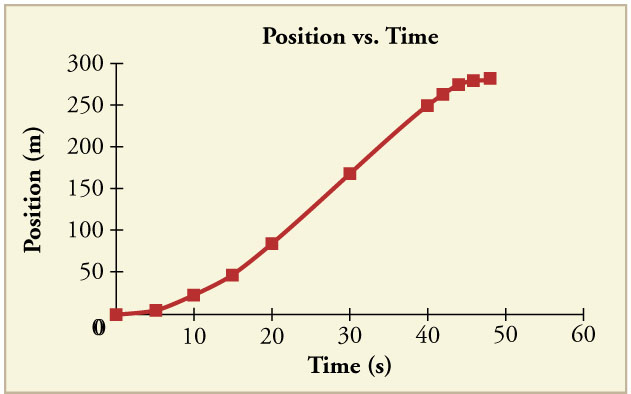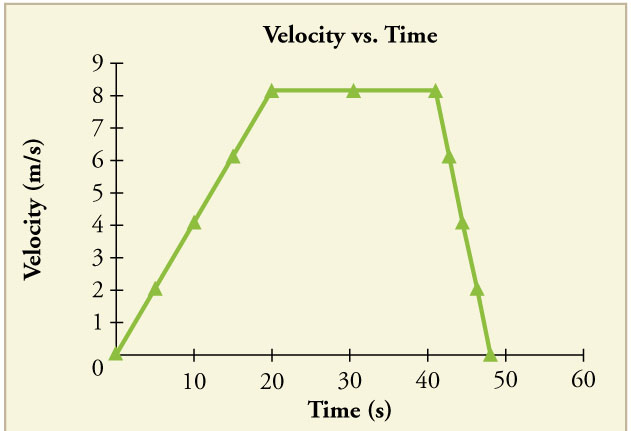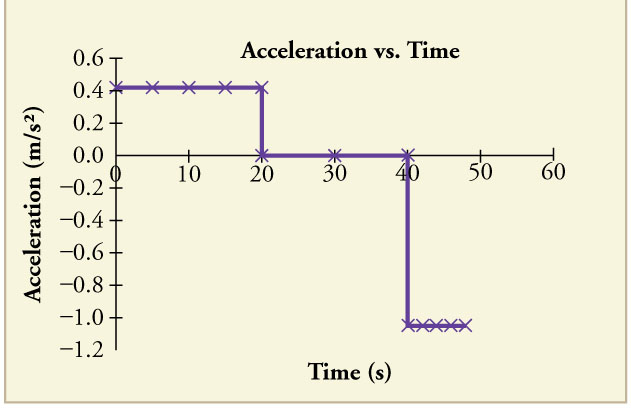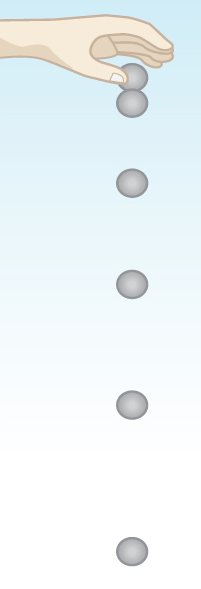# Graphical Interpretation

The graphical representation of acceleration over time can be derived through the graph of an object’s position over time.

### Learning Objectives

Distinguish the difference between how to plot a velocity graph and how to plot an acceleration graph

### Key Takeaways

#### Key Points

• Acceleration is the rate at which the velocity of a body changes with time.
• Acceleration is a vector that points in the same direction as the change in velocity, though it may not always be in the direction of motion.
• Because acceleration is velocity in m/s divided by time in s, we can derive a graph of acceleration from a graph of an object’s speed or position.

#### Key Terms

• acceleration: The amount by which a speed or velocity increases (and so a scalar quantity or a vector quantity).
• velocity: A vector quantity that denotes the rate of change of position with respect to time, or a speed with a directional component.
• position: A place or location.

In physics, acceleration is the rate at which the velocity of a body changes with time. It is a vector quantity with both magnitude and direction. Acceleration is accompanied by a force, as described by Newton’s Second Law; the force, as a vector, is the product of the mass of the object being accelerated and the acceleration (vector), or $\text{F}=\text{ma}$. The SI unit of acceleration is the meter per second squared: $\displaystyle \frac{\text{m}}{\text{s}^2}$

Acceleration is a vector that points in the same direction as the change in velocity, though it may not always be in the direction of motion. For example, when an object slows down, or decelerating, its acceleration is in the opposite direction of its motion.

The motion of an object can be depicted graphically by plotting the position of an object over time. This distance-time graph can be used to create another graph that shows changes in velocity over time. Because acceleration is velocity in $\displaystyle \frac{\text{m}}{\text{s}}$ divided by time in s, we can further derive a graph of acceleration from a graph of an object’s speed or position.

is a graph of an object’s position over time. This graph is similar to the motion of a car. In the beginning, the object’s position changes slowly as it gains speed. In the middle, the speed is constant and the position changes at a constant rate. As it slows down toward the end, the position changes more slowly. From this graph, we can derive a velocity vs time graph.Position vs Time Graph: Notice that the object’s position changes slowly at the beginning of the journey, then more and more quickly as it picks up speed. Its position then changes more slowly as it slows down at the end of the journey. In the middle of the journey, while the velocity remains constant, the position changes at a constant rate.

This shows the velocity of the object over time. The object’s velocity increases in the beginning as it accelerates at the beginning, then remains constant in the middle before it slows down toward the end. Notice that this graph is a representation of the slope of the previous position vs time graph. From this graph, we can further derive an acceleration vs time graph.Velocity vs Time: The object’s velocity increases as it accelerates at the beginning of the journey. It remains the same in the middle of the journey (where there is no acceleration). It decreases as the object decelerates at the end of the journey.

To do this, we would also plot the slope of the velocity vs time graph. In this graph, the acceleration is constant in the three different stages of motion. As we noted earlier, the object is increasing speed and changing positions slowly in the beginning. The acceleration graph shows that the object was increasing at a positive constant acceleration during this time. In the middle, when the object was changing position at a constant velocity, the acceleration was 0. This is because the object is no longer changing its velocity and is moving at a constant rate. Towards the end of the motion, the object slows down. This is depicted as a negative value on the acceleration graph. Note that in this example, the motion of the object is still forward (positive), but since it is decelerating, the acceleration is negative.Acceleration vs Time Graph: The object has positive acceleration as it speeds up at the beginning of the journey. It has no acceleration as it travels at constant velocity in the middle of the journey. Its acceleration is negative as it slows down at the end of the journey.

Graphing Motion: A brief introduction to particle diagrams and motion graphs.

## Motion with Constant Acceleration

Constant acceleration occurs when an object’s velocity changes by an equal amount in every equal time period.

### Learning Objectives

Describe how constant acceleration affects the motion of an object

### Key Takeaways

#### Key Points

• Assuming acceleration to be constant does not seriously limit the situations we can study and does not degrade the accuracy of our treatment.
• Due to the algebraic properties of constant acceleration, there are kinematic equations that can be used to calculate displacement, velocity, acceleration, and time.
• Calculations with constant acceleration can be done in relation to one-dimensional motion as well as two-dimensional motion.

#### Key Terms

• acceleration: The amount by which a speed or velocity increases (and so a scalar quantity or a vector quantity).
• kinematic: of or relating to motion or kinematicsOne-Dimensional Motion: When you drop an object, it falls vertically toward the center of the earth due to the constant acceleration of gravity.

An object experiencing constant acceleration has a velocity that increases or decreases by an equal amount for any constant period of time. Acceleration can be derived easily from basic kinematic principles. It is defined as the first time derivative of velocity (so the second derivative of position with respect to time):

$\displaystyle \text{a}= \frac {\partial \text{v}}{\partial \text{t}}=\frac {\partial ^2 \text{x}}{\partial \text{t}^2}$

Assuming acceleration to be constant does not seriously limit the situations we can study and does not degrade the accuracy of our treatment, because in a great number of situations, acceleration is constant. When it is not, we can either consider it in separate parts of constant acceleration or use an average acceleration over a period of time.

The motion of falling objects is a simple, one-dimensional type of projectile motion in which there is no horizontal movement. For example, if you held a rock out and dropped it, the rock would fall only vertically downward toward the earth. If you were to throw the rock instead of just dropping it, it would follow a more projectile-like pattern, similar to the one a kicked ball follows.

Projectile motion is the motion of an object thrown or projected into the air and is subject only to the acceleration of gravity. The object thrown is called a projectile, and the object’s path is called its trajectory. In two-dimensional projectile motion, there is both a vertical and a horizontal component.

Due to the algebraic properties of constant acceleration, there are kinematic equations that relate displacement, initial velocity, final velocity, acceleration, and time. A summary of these equations is given below.

$\text{x}=\text{x}_0+ \bar{\text{v}}\text{t}$

$\displaystyle \bar{\text{v}} = \frac{\text{v}_0 +\text{v}}{2}$

$\text{v} = \text{v}_0 + \text{at}$

$\displaystyle \text{x}= \text{x}_0+\text{v}_0\text{t}+ \frac{1}{2}\text{at}^2$

$\text{v}^2 = \text{v}_0^2 + 2\text{a}(\text{x}-\text{x}_0)$

Constant Acceleration Explained with Vectors and Algebra: This video answers the question “what is acceleration? “.